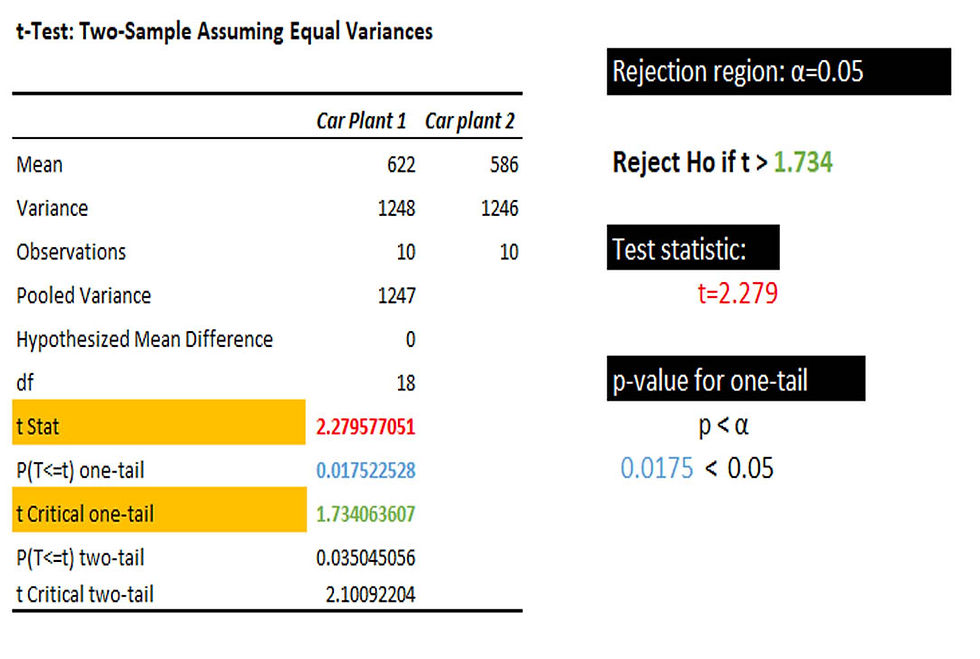top of page
Search
• Marek Vavrovic

# Hypothesis testing, T-Distribution.

• Hypothesis testing is just a method for testing a claim or hypothesis about a parameter in a population [mean, proportion]. In hypothesis testing we study the sample. Results of the sample are generalized to entire population.

• The “Null Hypothesis” denoted as H0 , this means testing a claim that already has some established parameters.

• The “Alternative Hypothesis” is denoted as H1, this is known as the research hypothesis. It involves the claim to be tested.

• Four steps of hypothesis testing are:

(I) We state the Hypothesis

(II) Set the criteria for a decision

(III) Compute the test statistic

(IV) Make a decision

Hypothesis testing is just a method for testing a claim or hypothesis about a parameter in a population, using data measured in a sample. The goal of hypothesis testing is to determine the likelihood that a population parameter, such as the mean (µ), is likely to be true.

The “Null Hypothesis” denoted as H0, this means testing a claim that already has some established parameters. The null hypothesis is always the accepted fact. It is a starting point. We test whether the value stated in the null hypothesis is likely to be true.

The “Alternative Hypothesis” is denoted as H1, this is known as the research hypothesis. It involves the claim to be tested. An alternative hypothesis (H0) is a statement that directly contradicts a null hypothesis by stating that that the actual value of a population parameter is less than, greater than, or not equal to the value stated in the null hypothesis. The alternate hypothesis is formulated depending on whether a one-tail or two-tail test is required:

Purpose of a t-test.

With the normal distribution we use z-scores and we must know the population’s standard deviation (σ) to calculate Z. But in the real world we often do not know the population standard deviation. Using the t-table, the t-test determines if there is a significant difference between two sets of data.

Due to variance and outliers, it is not enough just to compare mean values. A t-test also considers sample variances.

TYPES OF T-TEST

One sample t-test

Tests the null hypothesis that the population mean is equal to a specified value µ based on a sample mean.

Formula for t-statisticIndependent two-sample t-test

Test the null hypothesis that two sample means x1 and x2 are equal.

Example: We need to check if the mean test scores of 2 separate samples of students have a statistically significant difference.

The calculation of the t-statistic differs slightly for the following scenarios:

-equal sample size, equal variance

-unequal sample size, equal variance

-equal or unequal sample size, unequal variance

Dependent, paired-sample t-test

We use this type of t-test when the samples are dependent:

- One sample has been tested twice (repeated measurements)

- Two samples have been matched or “paired”

Example: We need to check of the same group of students has improved results on test scores before preparation course and after preparation course.

Example #1

We have daily production data over the same 10 days. Company wants to know if there is a significant difference in production between these to car plants.From this sample, it looks like Car Plat 1 produce 36 more cars per day than Car Plant 2. Is this statistically significant more amount of cars?

Ho: X1 <= X2, Null hypothesis

Ha: X1>X2, Alternative hypothesis

• one tailed test

You can compute the variance using VAR.S() function or Data Analysis > Descriptive Statistics. Our two samples have similar variances. I’m going to use t-Test: Two-Sample Assuming Equal Variances.Compare t-value (2.28) to the critical value (1.734): 2.28 > 1.73

Since our computed t-value is greater than the critical value, we reject the null hypothesis. We believe with 95% confidence that Car Plant 1 produces more cars per day than Car Plant B.

IF P-VALUE IS LOW P<=α, THE HULL HYPOTHESIS MUST GO [REJECT]Example #2: t-Test: Two-Sample

Do full time students spend more time studying stats than part time students. Test at α=0.05.

µf = population mean study time for full time students

µp = population mean study time for part time students

Ha: µf > µp Ha: µf - µp > 0 Alternative hypothesis

Ho: µf - µp <=0 Null hypothesis

Full time: 3.2 1.5 6.5 0.2 3.7 3.3 1.7 3.6 3.8 5.3 6.9 3.6 1.7 5.2 5.2 1.2 7.2 3.9 1.9 5.3

Part time: 3.1 3.4 4.6 2.8 2.3 1.5 3.8 9.5 4.3 2.7 1.6 1.6 3.2 4.2 3.9 1.2

Ha: µf - µp > 0, The alternative hypothesis says we must conduct right-tailed testConduct t-test.

1.2.The calculated t-value is OUTSIDE THE REJECTION REGION. And p-value > α. There is not enough evidence to conclude that full time students spend more time studying stats than part time students.

P(T<=t) one-tail > α

0.28 > 0.05

We fail to reject the null hypothesis: Ho: µf - µp <=0